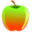CBEST Test Prep

# CBEST Math Test Tips - Page two.

### Mental Math Tips

Try a problem like 56 + 47. It has to be less than 60 + 50 or 110. We also know from the preceding example it must be larger than 56 + 40 or 50 + 47 (96 or 97). We should have already concluded the answer will end in a 3 because 6 + 7 = 13. The only number between 97 and 110 that ends in a 3 is 103. The answer MUST be 103.

The same principle holds true for subtracting. Try 244 - 17. The answer lies between 244 - 10 = 234 and 244 - 20 = 224. We know it ends with a 7 because 14 - 7 = 7. (Remember how numbers relate?) Therefore, the only number between 224 and 234 that ends in a 1 is 231. The answer MUST be 231.

Practice all of the above before going on.

If you were not convinced before, you now should agree that large calculations are quite simple. If you think logically.

How about much larger numbers? What about 756 - 234. Use the same principle. All that matters is 56 - 34 = 22. The answer will end with a 22. You should logically conclude that the first digit must be a 5 for an answer of 522.

Try   846 - 59. We know that 16 - 9 = 7, so the answer will end in a 7. The answer will be slightly more than 846 - 60 = 786. Because we have subtracted 1 more than we should have, we need to add 1 to get the correct answer of 787.

We can now work on splitting numbers up to do even more difficult calculations. We'll start with 1,235 + 2,486. We know we have 1,000 + 2,000 or 3,000. We also have 200 + 400 or 600. So far we know the answer is a little more than 3600. See how easy that was? All we need to do is to see how much more. Well, we also have 80 + 30 or 110. Now we have an even closer answer of 3600 + 110 or 3710. Just add the 5 + 6 to get the last piece. 6+ 5 = 11. Add 10 + 11 to get 21. The answer to 1,235 + 2486 is 3710 + 11 or 3721. But we should  have already had in out minds that the answer ends in a 1.

Let's try  a subtraction. Consider the problem 987 - 348. Isn't it the same as 900 - 300, then 87 - 48? We know the answer is 600 and something. We also know it ends with a 9 because 17 - 8 = 9. (Remember the part on relating small problems with large problems.) We should also recognize a boundary for 87 - 48. It will be close to 87 - 50, or 37. But I know it ends in a 9, so how about a guess of 39? I could also get 39 by realizing I have subtracted 2 more than I should have so I need to add 2 back. The answer to 987 - 348 must be 639. Notice how the 900 and the 300 became almost irrelevant to any calculations?

How about  924 - 786. Hmmmmmm. Slightly different because 24 is smaller than 86 and that involves a different thought process. But not really. I only need to think boundaries again. We should be able to rapidly do 924 - 800 in our heads to get 124. But I did not subtract enough away, so it must be a little more than 124. I also know the answer ends with an 8. (I'll let you remember why.) I should recognize some guesses. I know it ends with and 8 and is more than 124. I could guess 128 or 138 or...virtualluy anything that is given as a choice. Suppose the choices were:
a. 118
b. 128
c. 138
d. 148
e. 158
I think you can now pick c as the logical choice.

Let's try 924 - 786 using a different approach. I can look at intervals in the numbers given. I am looking for the interval between 924 and 786, right? Can you logically see it is equal to 24 + 100 + 14? The interval is 924 to 901, 900 to 801, 800 to 786.

How about 675 - 256. The interval (or answer) is 75 + 300 + 44. (675 to 601, 600 to 301, 300 to 256)
So, if you hate subtraction, change it to an addition problem!

It works for smaller calculations also. For example, 84 - 56. You should now recognize right off it is 4 + 20 + 4, or 28. Which leads to the following hint:

Don't count numbers you don't have too! Think logically as to what the are.
Think about the problem 107 - 100. Do I have to count out all 100 to subtract? No. I can do it in one block.

This is the same way we do 23 + 2. We do not count out 23 before we add the 2, do we? Of course not. We know it is 2 more than 23.

## More CBEST Test Prep Resources

CBEST Test Tips - Overall tips to take and pass the CBEST Test.

CBEST Math Tips - Read our tips to pass the math section of the CBEST Test.

Essay Writing Tips for the CBEST - Get our CBEST essay writing guide here.